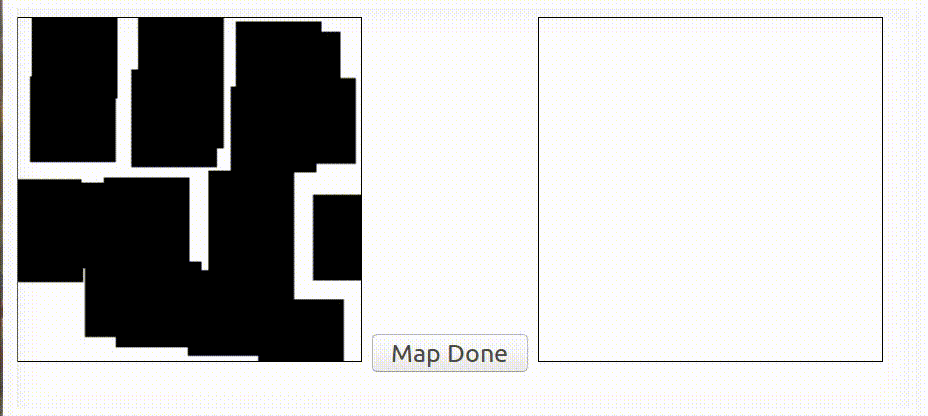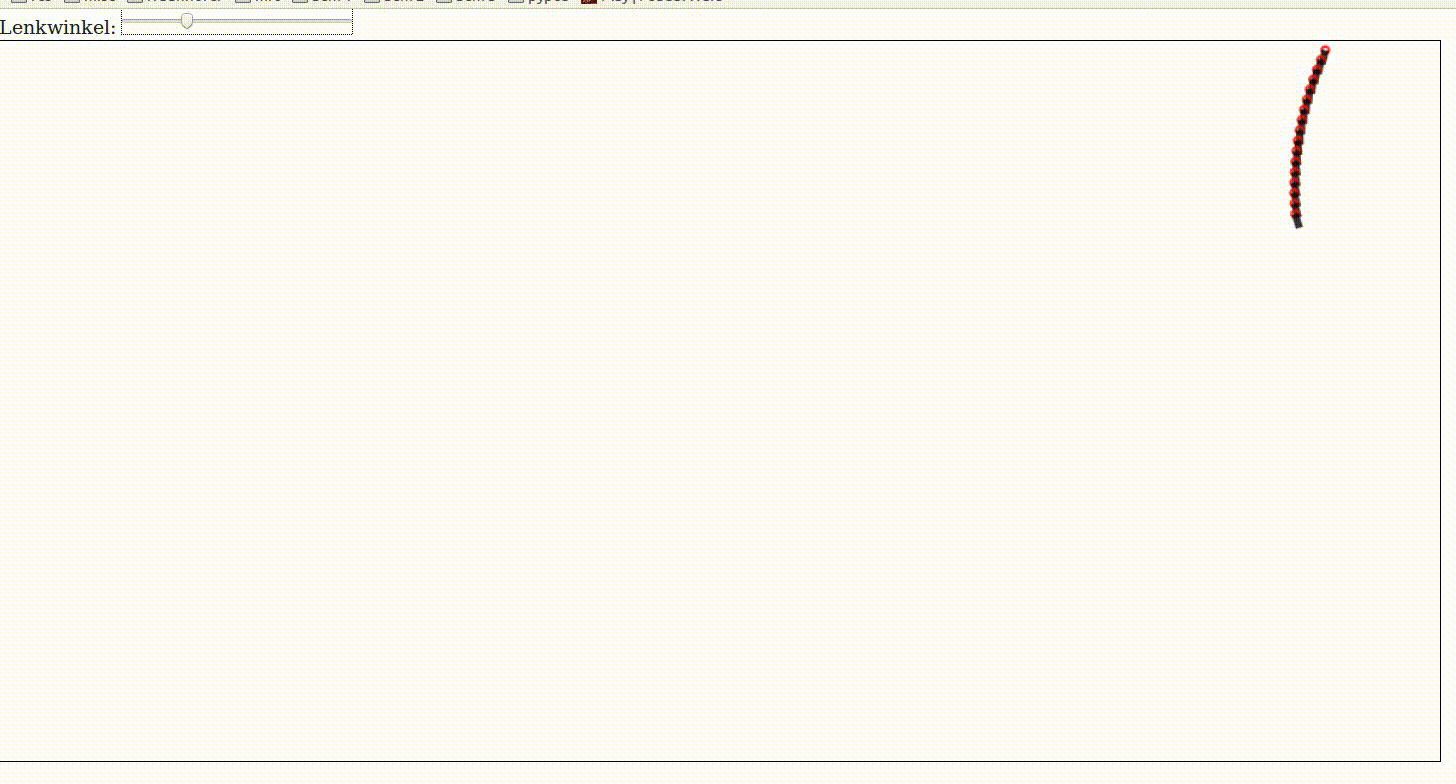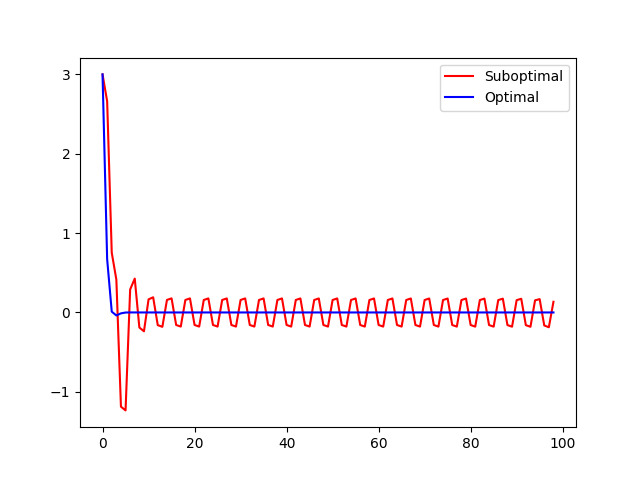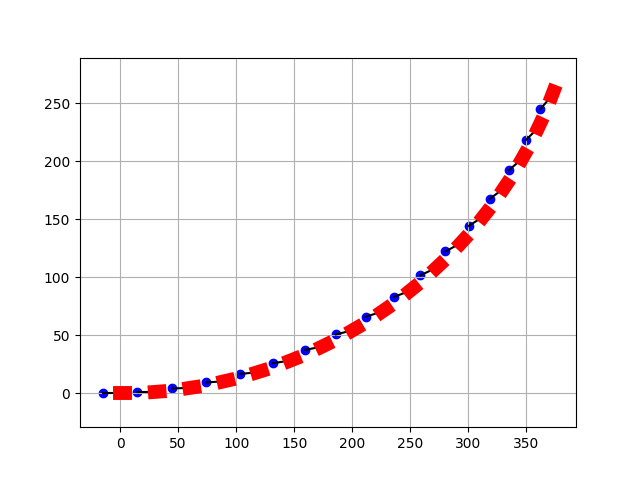# Generating Behavior for Vehicles

by Daniel Pollithy

This post is a loose collection of JavaScript demos I made while studying for the course “Generating Behavior for Vehicles”.

The following two gifs show you how two of the demos work:

**Planning a path through a city map with A star **A drifting car## Kinematic One Track Model

A car is controlled by its steering angle $\delta$ and its acceleration. If we want a vehicle to perform any kind of maneuver then we have to relate the input commands (steering and acceleration) with future positions of the car.

For this purpose we use dead reckoning. In the following example we assume to have a car with constant velocity. You can control its steering angle with the slider below. It starts to drive once you change the steering angle a little bit.

The model is called kinematic because we only model the geometry and not the dynamics. This will be our next step. It is called One-Track because we assume that the two wheels on both axis can be modelled by averaged wheels in the center of both axis.

The simple differential equations are:

$\dot{x} = v \cdot \frac{\cos(\delta)}{l}$ $\dot{y} = v \cdot \frac{\sin(\delta)}{l}$ $\dot{\theta} = v \cdot \frac{\tan(\delta)}{l}$

Assuming constant inputs we use the Forward Euler to calculate the new state:

$x = x + \Delta x$ $y = y + \Delta y$ $\theta = \theta + \Delta \theta$

This model is in general unrealistic because it assumes that the car can change its direction instantaneously. But it works good enough as long as the velocity of the car is low. Therefore we could use it to build a parking assistant.

## Linearized Dynamic One Track Model

Now we consider a car which is driving with a regular speed like 50 km/h. In this model we accept that there can be a difference between the steering angle and the real angle in which direction the mass of the vehicle moves. Usually we want this discrepancy to be as low as possible because it is difficult to control the car then. Think of a drifting car.

In the following demo you can control the steering angle again (velocity is constant). Because it is easy to loose control and leave the screen, the car will come back into the screen if that happens.

This linearized dynamic one track model can be used in ESP systems to estimate the yaw rate of the car. If it is higher than the driver might expect it then it is becoming dangerous because the control over the car can be lost easily. Starting from this the ESP regulator can break tires or accelerate others to reduce the yaw rate to keep the car controllable.

ToDo: Add equations for dynamic one track model and show how to linearize

## Planning

We want our car to perform a maneuver. But what should it actually do? Let’s think about the scenario when a car wants to drive from one point in a city to another point. We assume that we have an HD (high definition) city map and we want to plan an optimal path.

First we should discretize the world to make the planning feasible and assume that the robot is a point:

• building a Voronoi graph around the obstacles
• polygon fitting into the free room
• Growing a tree structure into the space (Quad/Octree, something like Rapidly Exploring Random Trees, although they already search)

Then we convert the problem into a graph search with Dijkstra or A. This results in a trajectory. In the following demo every pixel is a node in the graph. You can click on the left canvas to draw obstacles (black). The robot starts in the left upper position and wants to drive to the right lower corner. It will search for the shortest path with A. All the expanded pixels are colored in blue.

## Optimal control

Given the trajectory from the last step, how do we get the steering angle and acceleration necessary to drive it?

One option would have been to plan in a Markov Decision Process. Instead of assuming a point robot we could frame it as follows:

Our car is in an initial state (steering angle, acceleration, position). It can take actions (steer and accelerate) which leads to different states. The different actions (lets assume they are discrete) lead to new states which we calculate using the motion model from before.

The different action values determine the branching factor of our state tree. Using the Bellman optimality equation we could iterate backwards starting at the goal in order to determine the “goodness” of each state at different time steps. Once the “goodness” has converged (the order of the values doesn’t change anymore or the values of the values either) we can greedily search through the graph in order to maximize the “goodness” at every time step.

This is called value iteration. Actually Dijkstra and A* are also based on the Bellman Recursion but the time index is replaced by the sorting index of the next expansion candidate. Dijkstra sorts by cost-so-far and A* by cost-so-far plus cost-to-go (a heuristic which has to be admissible, which means that it underestimates the real cost-to-go).

The problem with this discrete approach is that it would happily jump between accelerations and steering angles although in reality these values are continuous.

No matter where our target trajectory comes from and the controls for that we somehow have to “smoothen” them. We want to optimize them. Imagine the trajectory makes an abrupt corner. To directly follow it we would have to stop at the corner turn the wheels and accelerate again. We note that there is a trade off between following the trajectory and following the control inputs.

Finding the optimal solution depends on what we want. We can formulate a cost functional (a functional is a function of functions) which penalizes both deviations of the trajectory and the input controls. According to our needs we add weightings to the parts to balance it or stress that we have to reach the target.

The cost functional look as follows:

$J = x^T(k_f) Q_f x(k_f) + \sum_{k=1}^{k_{f}-1} x^T(k) Q_f x(k) + \sum_{k=0}^{k_{f}-1} u^T(k) R_f u(k)$

$Q_f$ is the weighting of the final state deviation. $Q$ is the weighting of the state deviation. $R$ is the weighting of the control input deviation.

We can solve this problem by applying the Bellman Recursion Formula backwards. But this time the amount of control values and state values is infinite because we are in a continuous domain. The cost functional between two time steps is a quadratic equation dependent on a weighting matrix P. We differentiate and solve for P which gives us the optimal P(k) for the time step k. We repeat this process until we reach the beginning. The formula to calculate P(k) is a Riccati difference equation which can be solved analytically.

Using P(k) we can calculate the optimal Gain matrix K* which we use to calculate the optimal control input u(k) = -K(k) x(k) for every time step.

If we don’t have a fixed target at time $k_f$ and our system is controllable and the system is time invariant (really?) then we can find an optimal P(k) which is optimal for all time steps. We call is $P_{\infty}$ and obtain it by solving the riccati differences equation until convergence. This leads to a time independent optimal control u* = -K* x(t) which is the LQR (linear quadratic regulator). As opposed to the classical creation of a regulator by determining eigenvalues, the advantages of the LQR are intuitive weighting with Q/R and no additional unwanted degrees of freedom.

ToDo: Clean this up and add the formulas.

Unfortunately I don’t have JavaScript example for this because I could not find a nice matrix package which helps me, therefore I will show some python code.import numpy as np
import matplotlib.pyplot as plt

def build_batch_matrices(A, B, k_f):
# Batch Matrices
A_batch = np.vstack( [ np.linalg.matrix_power(A, n) for n in range(0, k_f) ] )

B_batch = []

for k in range(1, k_f+1):
# build row
row = []
for m in range(0, k-1):
row.append(np.linalg.matrix_power(A, k-m-1) @ B)
row.append(B)

while len(row) < k_f:
row.append(np.zeros(B.shape))

B_batch.append(np.hstack(row))

B_batch = np.vstack(B_batch)

return A_batch, B_batch

def build_batch_cost(Q, R, k_f):
Q_batch = []
R_batch = []

for k in range(0, k_f):
# build row
row_q = []
row_r = []

for k2 in range(0, k_f):
if k == k2:
row_q.append(Q)
row_r.append(R)
else:
row_q.append(np.zeros(Q.shape))
row_r.append(np.zeros(R.shape))

Q_batch.append(np.hstack(row_q))
R_batch.append(np.hstack(row_r))

Q_batch = np.vstack(Q_batch)
R_batch = np.vstack(R_batch)

return Q_batch, R_batch

def build_H_F(A_batch, B_batch, Q_batch, R_batch, k_f):
H_batch = B_batch.T @ Q_batch @ B_batch + R_batch
F_batch = A_batch.T @ Q_batch @ B_batch

return H_batch, F_batch

# how many time steps
k_f = 100

# target trajectory
x = np.arange(0, 100, 1)
y = np.zeros(x.shape)
# different values for y are more interesting
# y = np.sin(x)

x_traj = np.stack([x,y]).T
kappa = np.zeros(x.shape)

A = np.array(
[
[1, 1],
[0, 1]
]
)

B = np.array(
[
[0, 0],
[0, 1]
]
)

# x(k+1) = A x(k) + B u(k)
# u(k) = [u, u]

# Riccati

# Q_f = P(k_f)
Q_f = np.array(
[
[100, 0],
[0, 1]
]
)

#  Q
Q = np.array(
[
[10, 0],
[0, 1]
]
)

#  R
R = np.array(
[
[1, 0],
[0, 1]
]
)

Ps = [Q_f]
Ks = []

# Loese Riccati-Differenzengleichung rückwärts
for k in reversed(range(0, k_f-1)):
P_kp1 = Ps[-1]
P_k = Q + A.T @ P_kp1 @ A - A.T @ P_kp1 @ B.T @ np.linalg.pinv( B.T @ P_kp1 @ B.T + R) @ B.T @ P_kp1 @ A.T
K_k = np.linalg.pinv( B.T @ P_kp1 @ B.T + R) @ B.T @ P_kp1 @ A.T
Ps.append(P_k)
Ks.append(K_k)

Ps = Ps[::-1]
Ks = Ks[::-1]

# Optimale Stellgroesse u(k) = -K(k) * x(k)

# state space
x_0 = np.array([
3, # d
0, # delta_psi
])

X = [x_0]

# euclidean
X_world = [(0, 3)]

# -------------------------
# The following lines contain a naive regulator which does not work as good
# as the riccati regulator
us = []

for k in range(0, k_f-1):
u_k = -Ks[k] @ X[k]

u_k = max(-np.pi/2.0, min(u_k, np.pi/2.0))

us.append(u_k)

# next time step
x_p = A @ X[k] + B @ u_k
# normalize delta_psi
# x_p = ((x_p + np.pi ) % 2*np.pi) - np.pi

# print(x_traj[k].copy())
x_world = x_traj[k].copy()
x_world += 0
x_world += x_p
X_world.append(x_world)

x_n = x_world - x_traj[k]
# x_n += np.random.normal(0, 0.01) # add some randomness

X.append(x_p)

# end of suboptimal regulator
# ----------------------------

A_batch, B_batch = build_batch_matrices(A, B, k_f-1)

us = np.hstack(us).T

x_batch_traj = A_batch @ x_0 + B_batch @ us

# u.T H u + 2 x_0.T F u

Q_batch, R_batch = build_batch_cost(Q, R, k_f-1)
H_batch, F_batch = build_H_F(A_batch, B_batch, Q_batch, R_batch, k_f-1)

u_opt = -np.linalg.inv(H_batch) @ F_batch.T @ x_0
x_opt_traj = A_batch @ x_0 + B_batch @ u_opt

plt.plot(x_batch_traj.reshape([-1, 2])[:, 0], label="Suboptimal", color="red")
plt.plot(x_opt_traj.reshape([-1, 2])[:, 0], label="Optimal", color="blue")

plt.legend()
plt.show()



## Geometric modeling of a trailer

The following simulation models a car with an attached trailer. This is a differential equation grounded on geometric considerations.import numpy as np
import matplotlib.pyplot as plt
import matplotlib.patches as patches

timesteps = 500

# variables
delta = 0.1 # steering angle

time = 0

# constants
car_length = 4
car_width = 2
trailer_length = 2
distance_car_trailer_axis = 15

x_start = 0

plot_dt = 30

# differential equations
def forward_euler(psi, v, gamma, delta_t=0.1):
psi_dot = v * np.tan(delta) * (1.0/car_length)
v_dot = 0.0
gamma_dot = v * np.tan(psi-gamma) * (1.0/distance_car_trailer_axis)

return [psi + delta_t*psi_dot,  v + delta_t*v_dot, gamma + delta_t*gamma_dot ]

def calc_pos(state, last_pos):
psi = state
v = state
x = last_pos
y = last_pos

x_new = x + np.cos(psi) * v
y_new = y + np.sin(psi) * v

return [x_new, y_new]

def calc_trailer_pos(state, last_pos):
gamma = state
x = last_pos
y = last_pos

return [x - np.cos(gamma) * distance_car_trailer_axis, y - np.sin(gamma) * distance_car_trailer_axis ]

state_traj = [
# psi, v, gamma
[0.0, 1.0, 0.0]
]

pos_traj = [
# x, y
[x_start, 0],
]

trailer_traj = [
[x_start - distance_car_trailer_axis, 0]
]

for t in range(timesteps):
state_traj.append(forward_euler(*state_traj[-1]))
pos_traj.append(calc_pos(state_traj[-1], pos_traj[-1]))
trailer_traj.append(calc_trailer_pos(state_traj[-1], pos_traj[-1]))

pos_traj = np.array(pos_traj)
trailer_traj = np.array(trailer_traj)

fig,ax = plt.subplots(1)

for i, row in enumerate(pos_traj):
if i%plot_dt != 0: continue
x_vec = [trailer_traj[i], pos_traj[i]]
y_vec = [trailer_traj[i], pos_traj[i]]

car_x = pos_traj[i, 0]
car_y = pos_traj[i, 1]
psi = state_traj[i]

# deichsel
ax.plot(x_vec, y_vec, 'k-')

# car
ax.plot([car_x, car_x + np.cos(psi) * car_length], [car_y, car_y + np.sin(psi) * car_length],  color="red", linewidth=10)

# trailer
ax.scatter(trailer_traj[i, 0], trailer_traj[i, 1], label="trailer", color="blue")

print(state_traj[-1])

plt.axis("equal")
# plt.legend()
plt.grid(True)

plt.show()